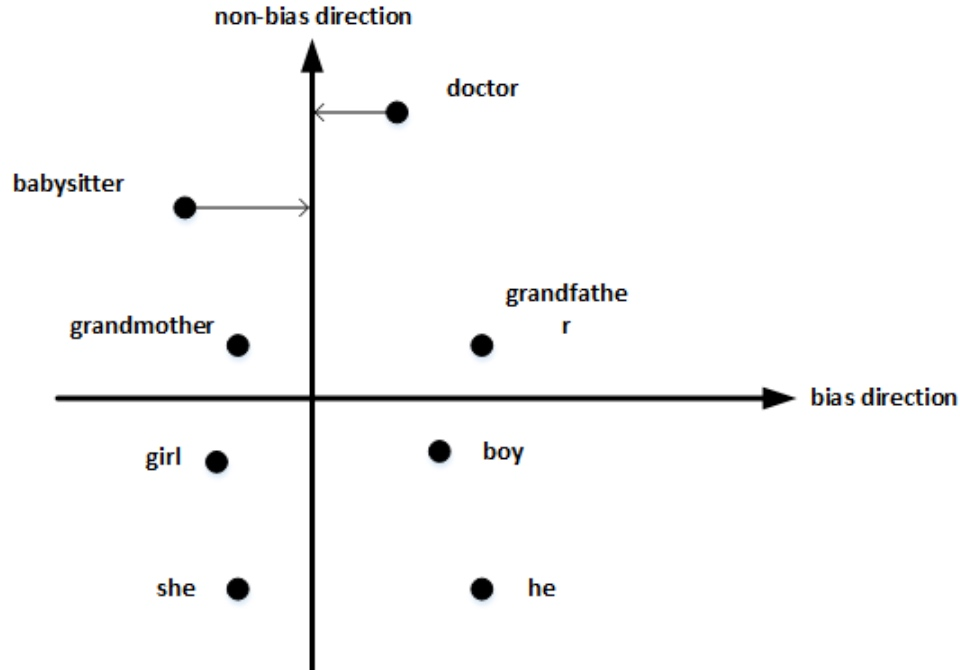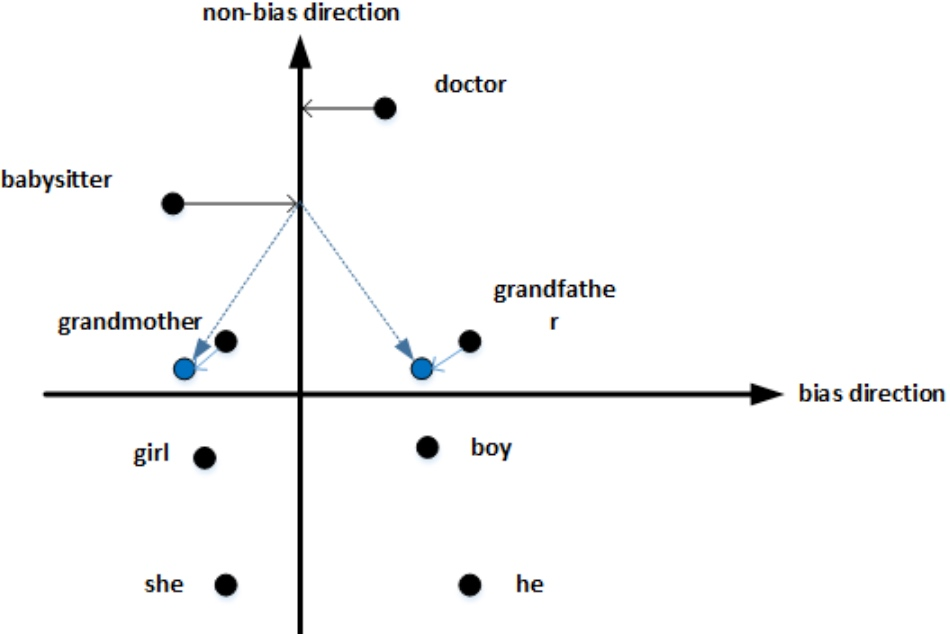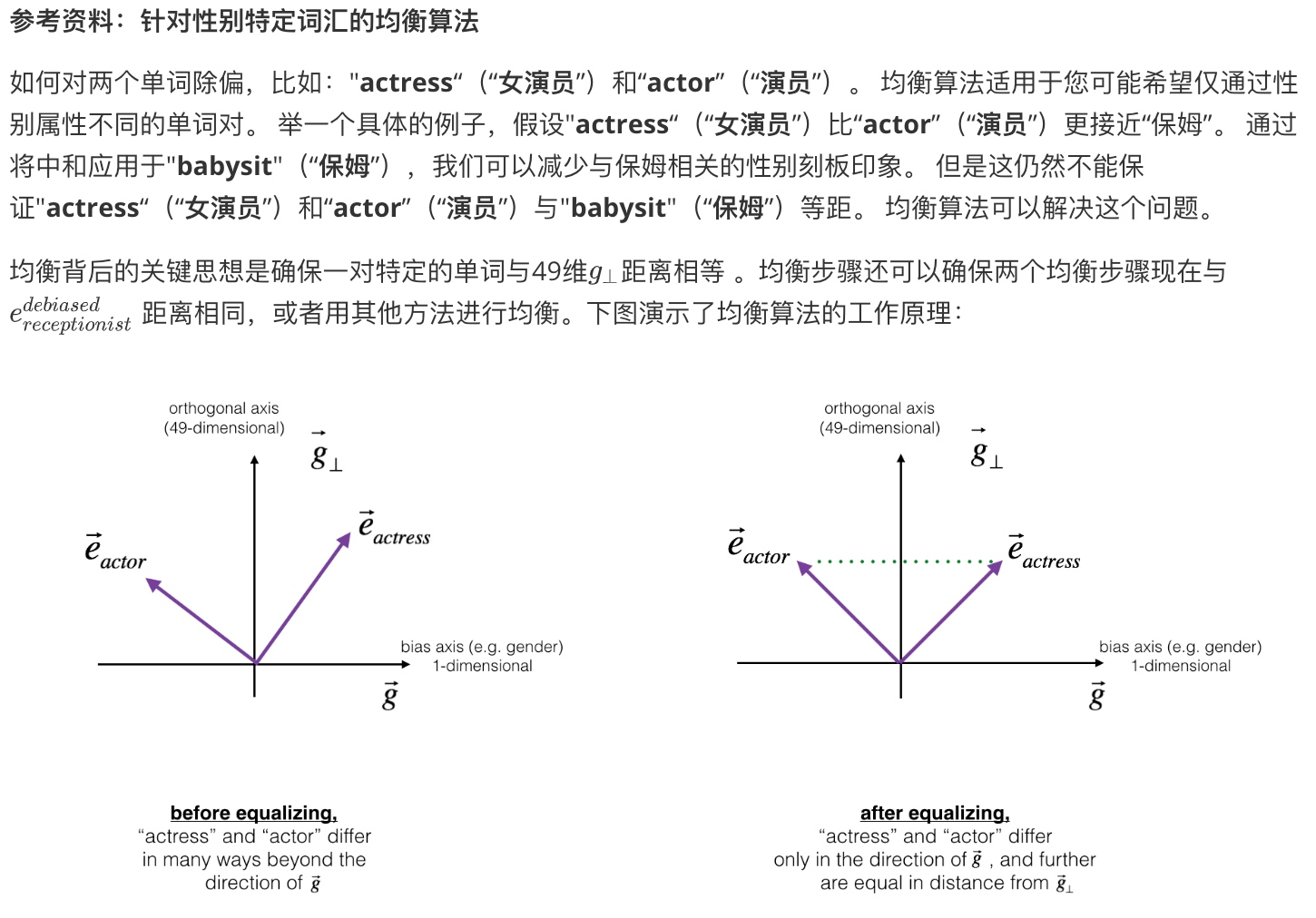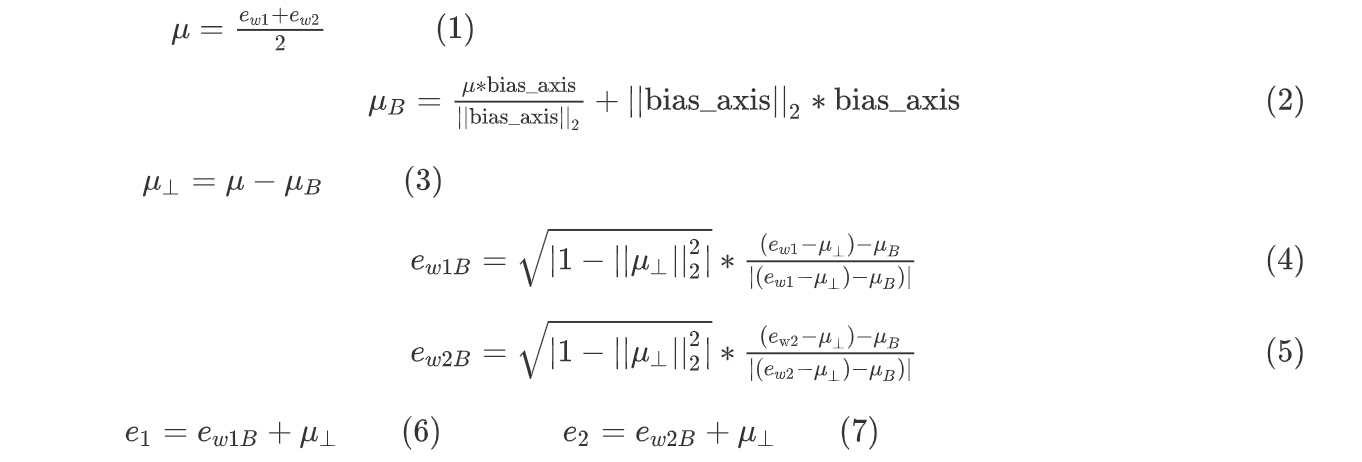views 689 words

## 词汇表征（Word Representation）

(Weakness of one-hot representation is that it treats each word as a thing onto itself and it doesn’t allow algorithm easily generalize the cross words)

one-hot表征单词的方法最大的缺点就是每个单词都是独立的、正交的，无法知道不同单词之间的相似程度, 这样使得算法对相关词的泛化能力不强. 例如

“I want a glass of orange juice”

“I want a glass of apple ___”

Apple和Orange都是水果，词性相近，但是单从one-hot编码上来看，内积为零，算法无法知道二者的相似性. 在NLP中，我们更希望能掌握不同单词之间的相似程度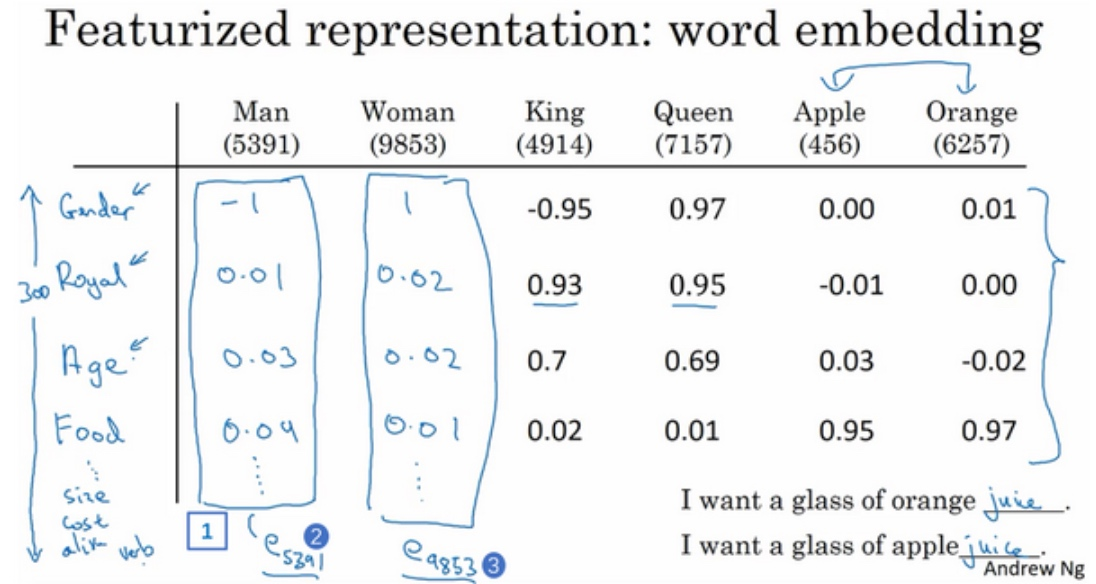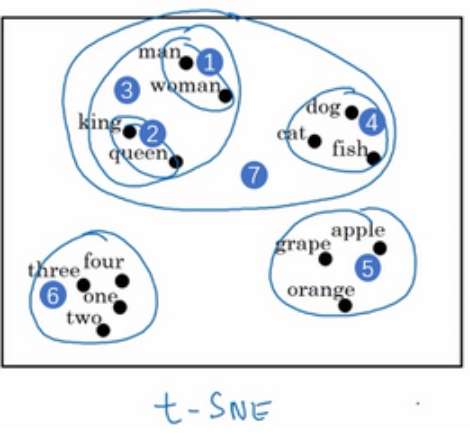## 使用词嵌入（Using Word Embeddings）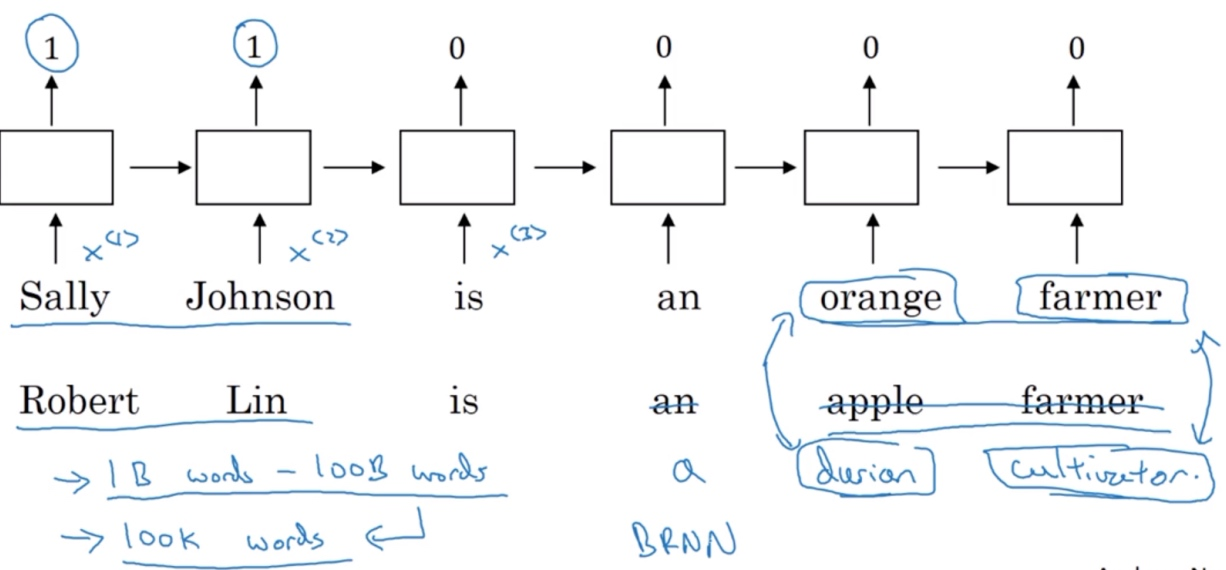‘Robert Lin is an apple farmer’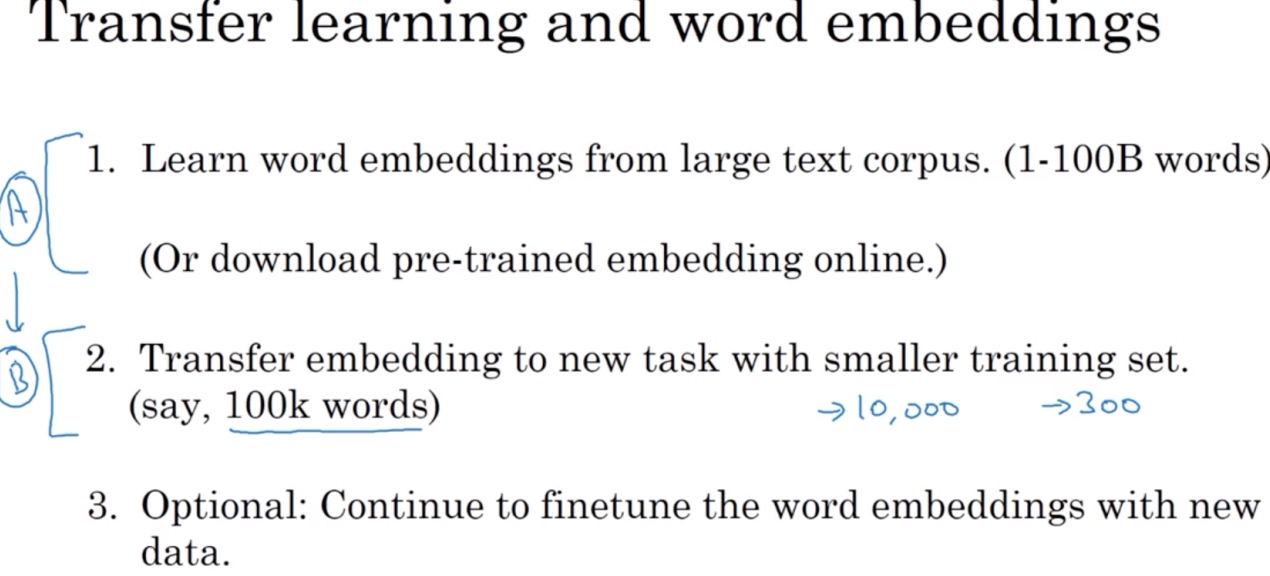featurized representation的特性使得很多NLP任务能方便地进行迁移学习. 词嵌入做迁移学习的步骤:

1. 从大量的文本集中学习word embeddings, 即所有单词的特征向量.
• 或者从网上下载预训练好的word embeddings
2. 使用较少的训练样本, 将word embeddings迁移到新的任务中.
• 这样就可以用更低维度的特征向量代替原来的10000维的one-hot向量
3. (optional)继续使用新数据微调word embeddings.
• 建议仅当训练样本足够大的时候，再进行上述第三步.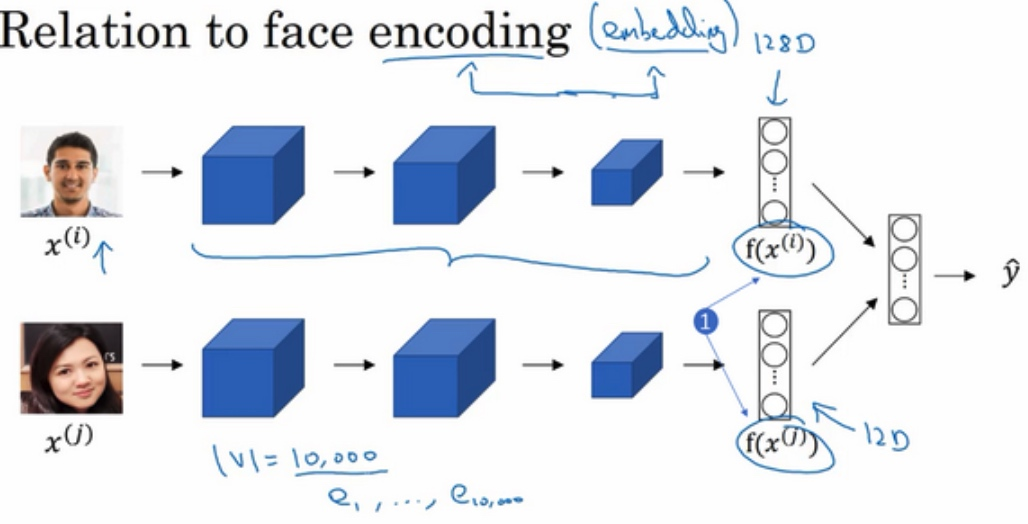## 词嵌入的特性（Properties of Word Embeddings）

Word embeddings可以帮助找到不同单词之间的相似类别关系.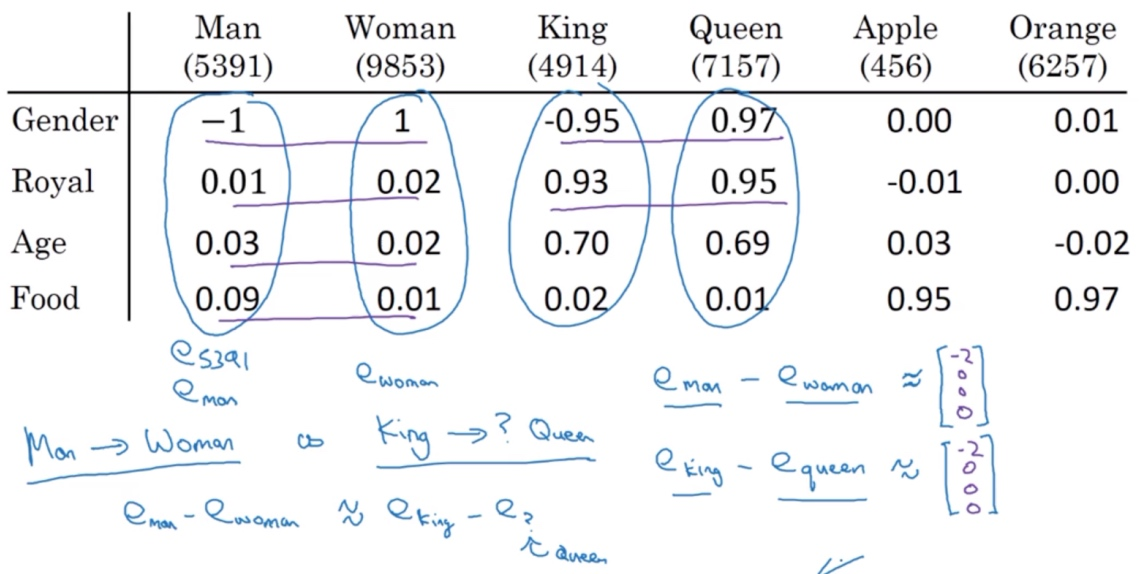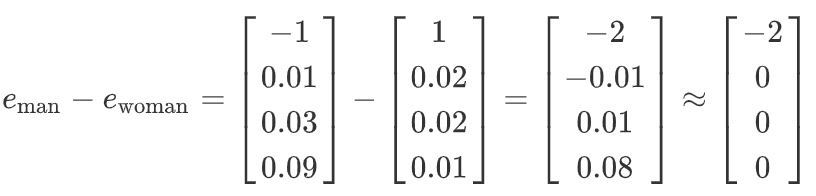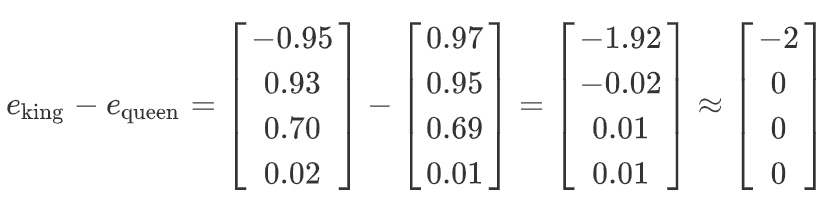[Mikolov T, Yih W T, Zweig G. Linguistic regularities in continuous space word representations[J]. In HLT-NAACL, 2013.]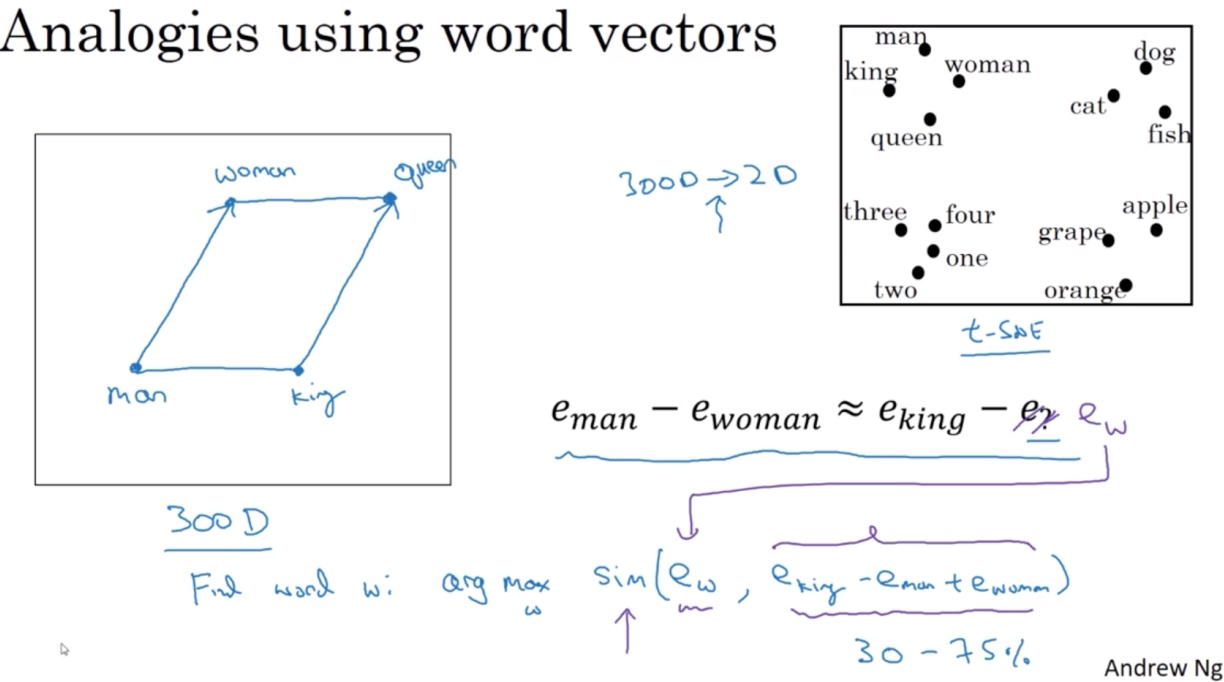$\mathop{argmax}_{?}{ (e_{?}, e_{king}-e_{man}+e_{woman}) }$ 这种方法来做类比推理准确率大概只有30%~75%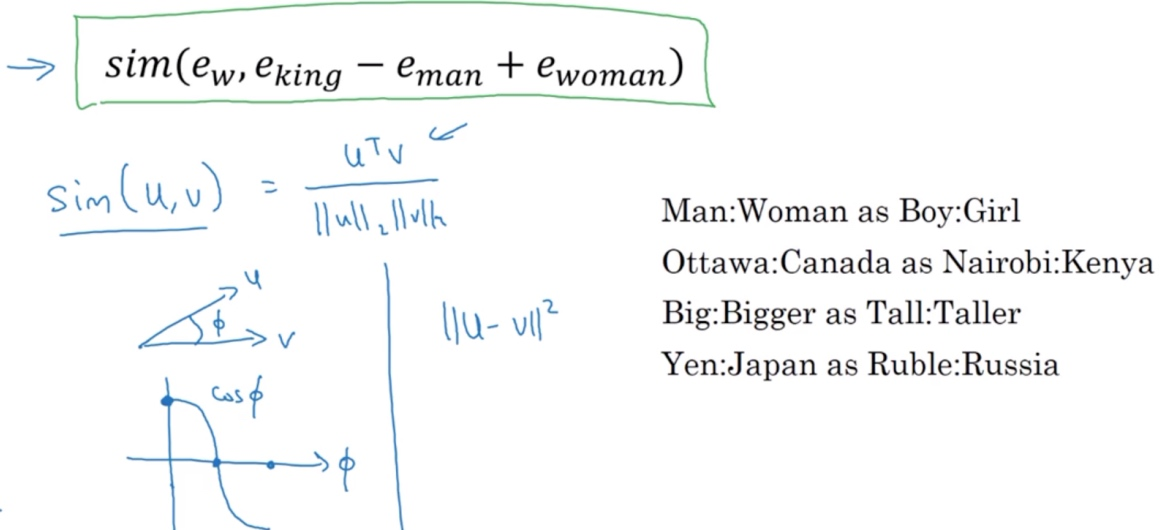$Sim(u,v)=\frac{u^Tv}{||u||\cdot ||v||}$

(分子其实就是u和v的内积, 如果u和v非常相似，那么它们的内积将会很大)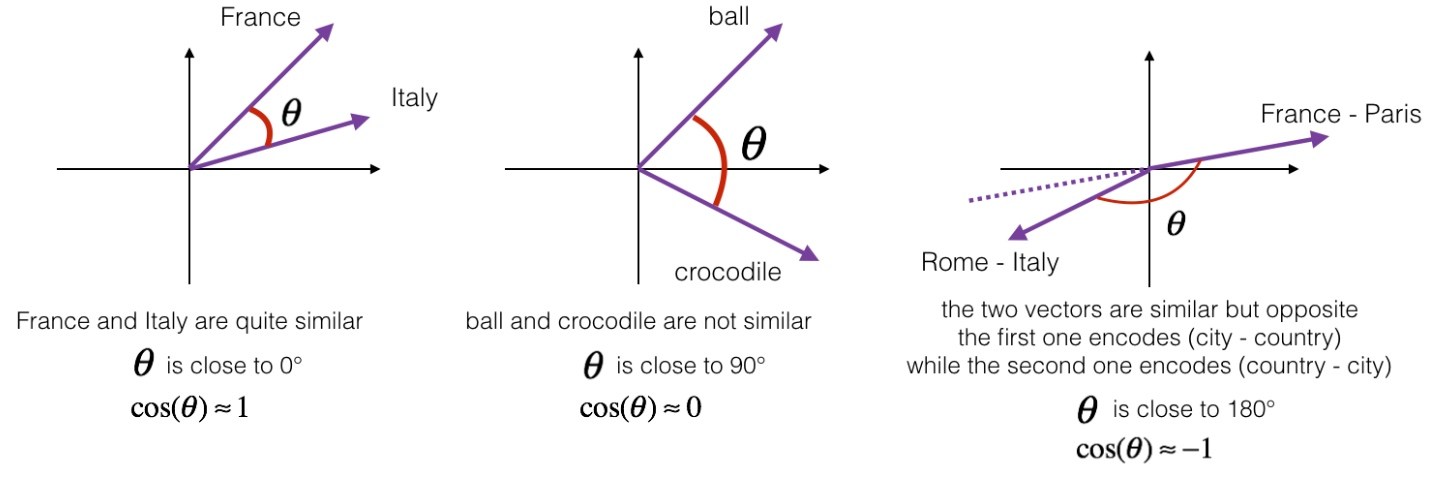## 嵌入矩阵（Embedding Matrix）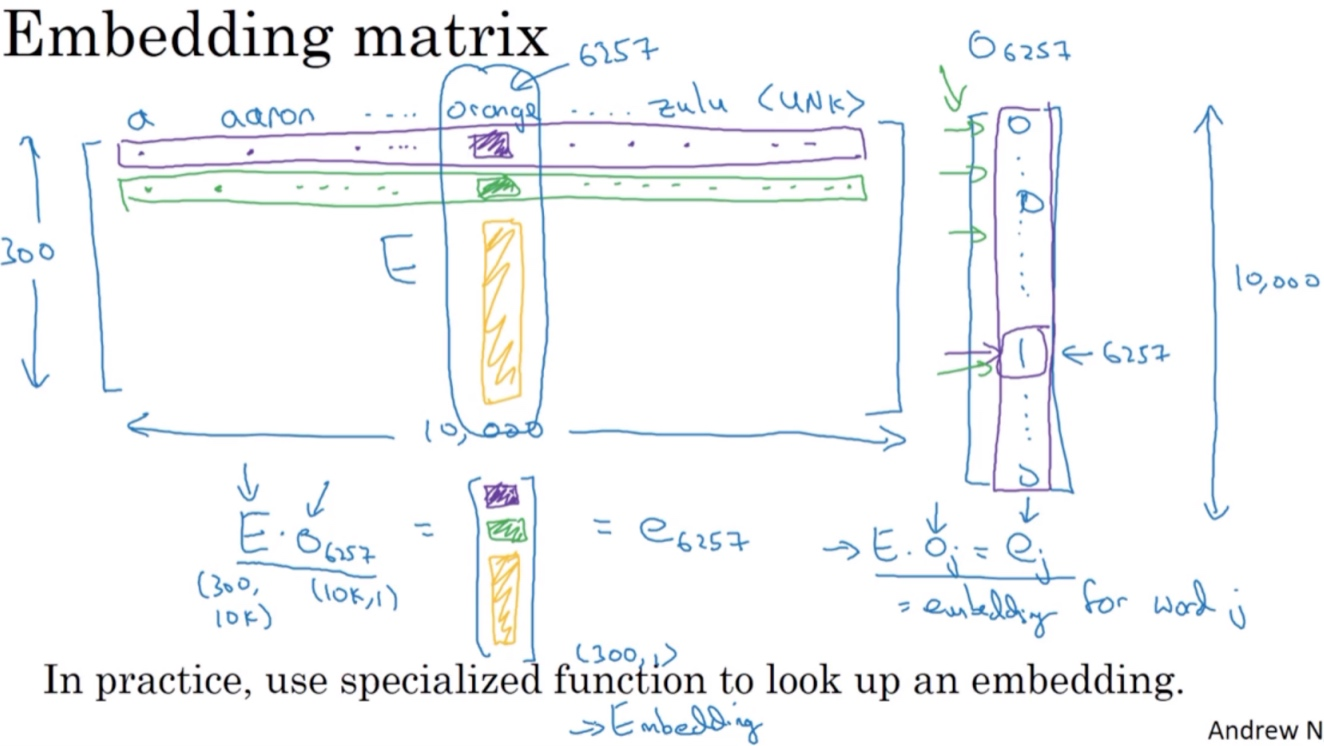$e_w=E\cdot O_w$

## 学习词嵌入（Learning Word Embeddings）

I want a glass of orange (juice).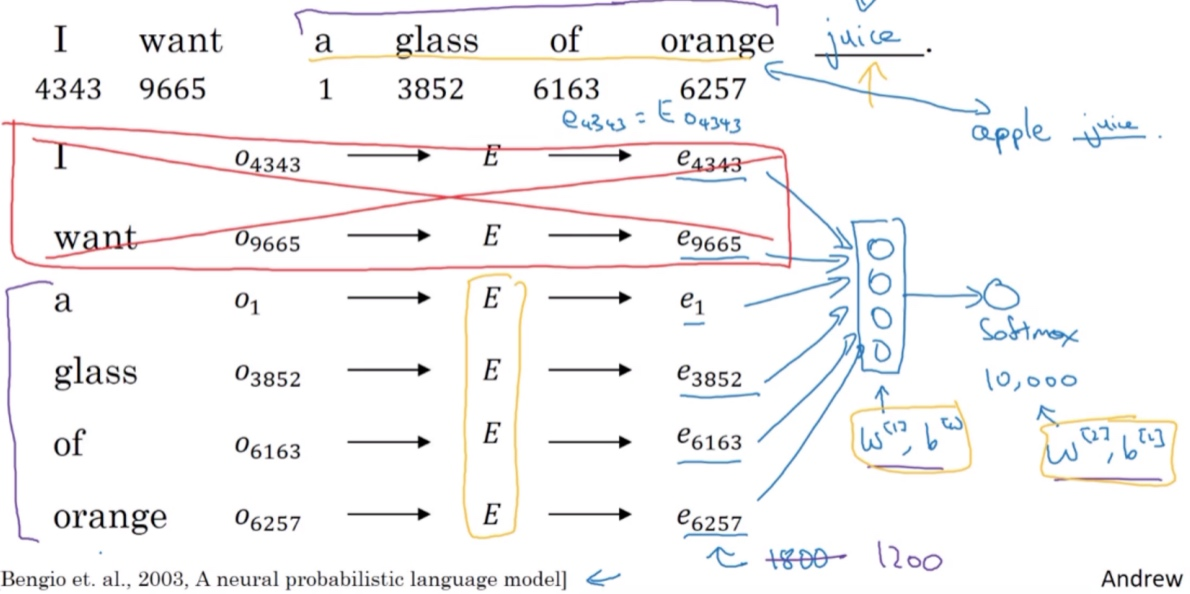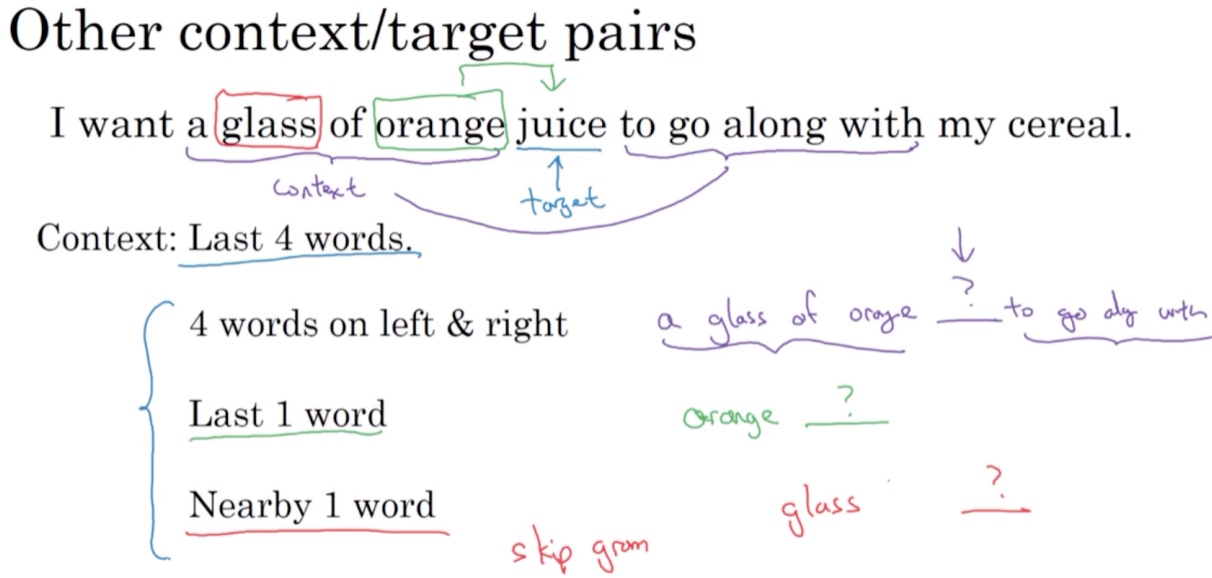• context: a glass of orange
• target: juice

## Word2Vec

[Mikolov T, Chen K, Corrado G, et al. Efficient Estimation of Word Representations in Vector Space[J]. Computer Science, 2013]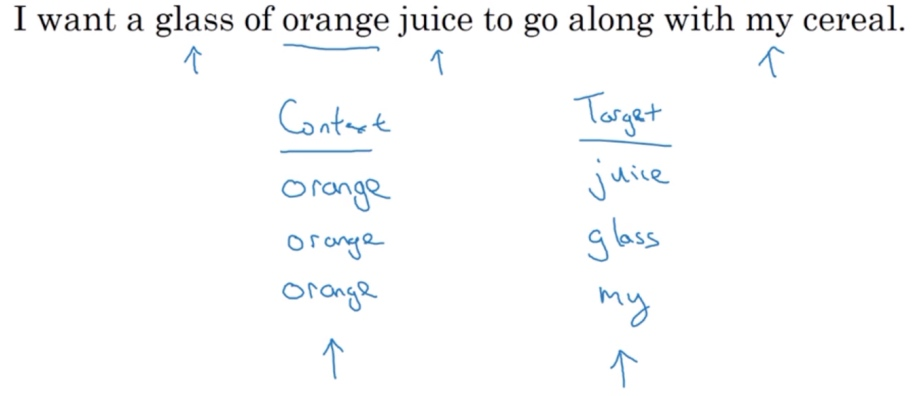‘I want a glass of orange juice to go along with my cereal.’

Skip-Gram模型的做法是：首先随机选择一个单词作为context, 例如’orange’; 然后使用一个宽度为5或10(自定义)的滑动窗, 在context附近选择一个单词作为target, 可以是’juice’, ‘glass’,… 最终得到了多个context—target对作为监督式学习样本.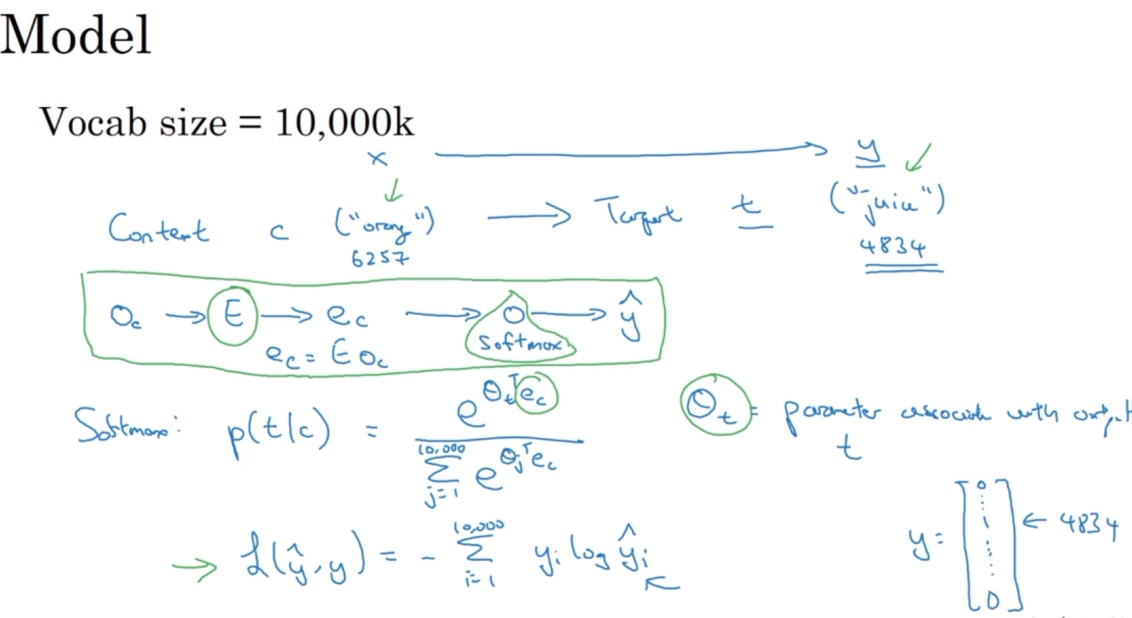1. 输入上下文词orange的one-hot向量$O_c$
2. 拿嵌入矩阵E乘以向量$O_c$, 得到输入的上下文词的嵌入向量$e_c \;\; (e_c = E \cdot O_c)$
3. 把向量$e_c$喂入一个softmax单元, 经过softmax单元的输出为： $\hat y = p(t|c)=\frac{e^{\theta_t^T\cdot e_c}}{\sum_{j=1}^{10000}e^{\theta_j^T\cdot e_c}}$

$L(\hat y,y)=-\sum_{i=1}^{10000}y_ilog\ \hat y_i$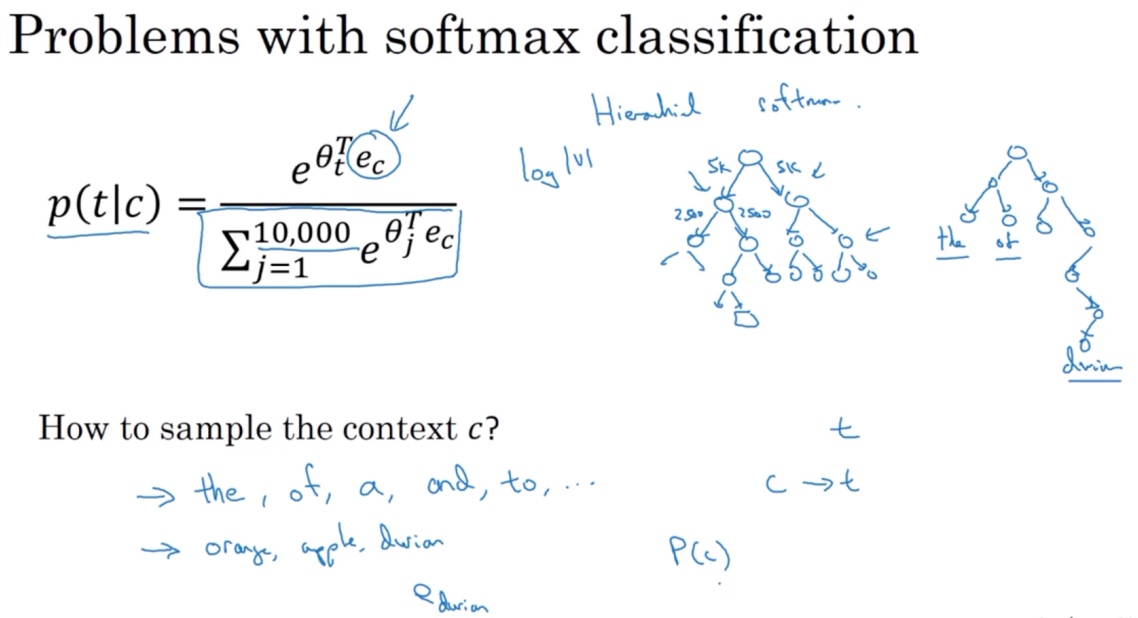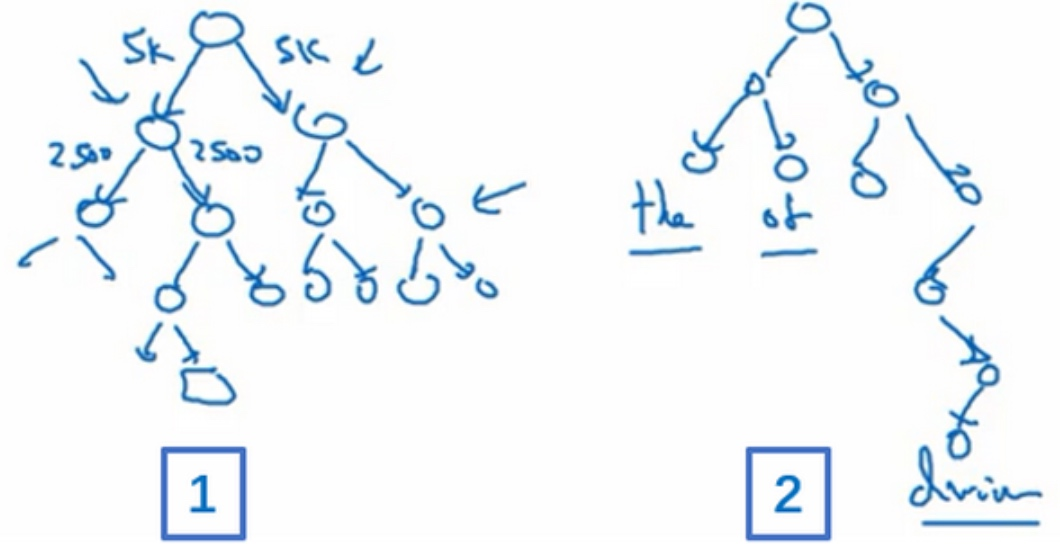Skip-Gram模型是Word2Vec的一种, Word2Vec的另外一种模型是连续词袋模型CBOW（Continuous Bag of Words), 它获得中间词两边的的上下文，然后用周围的词去预测中间的词，这个模型也很有效，也有一些优点和缺点.

CBOW是从原始语句推测目标字词；而Skip-Gram正好相反，是从目标字词推测出原始语句. CBOW对小型数据库比较合适，而Skip-Gram在大型语料中表现更好

## 负采样（Negative Sampling）

[Mikolov T, Sutskever I, Chen K, et al. Distributed Representations of Words and Phrases and their Compositionality[J]. 2013, 26:3111-3119.]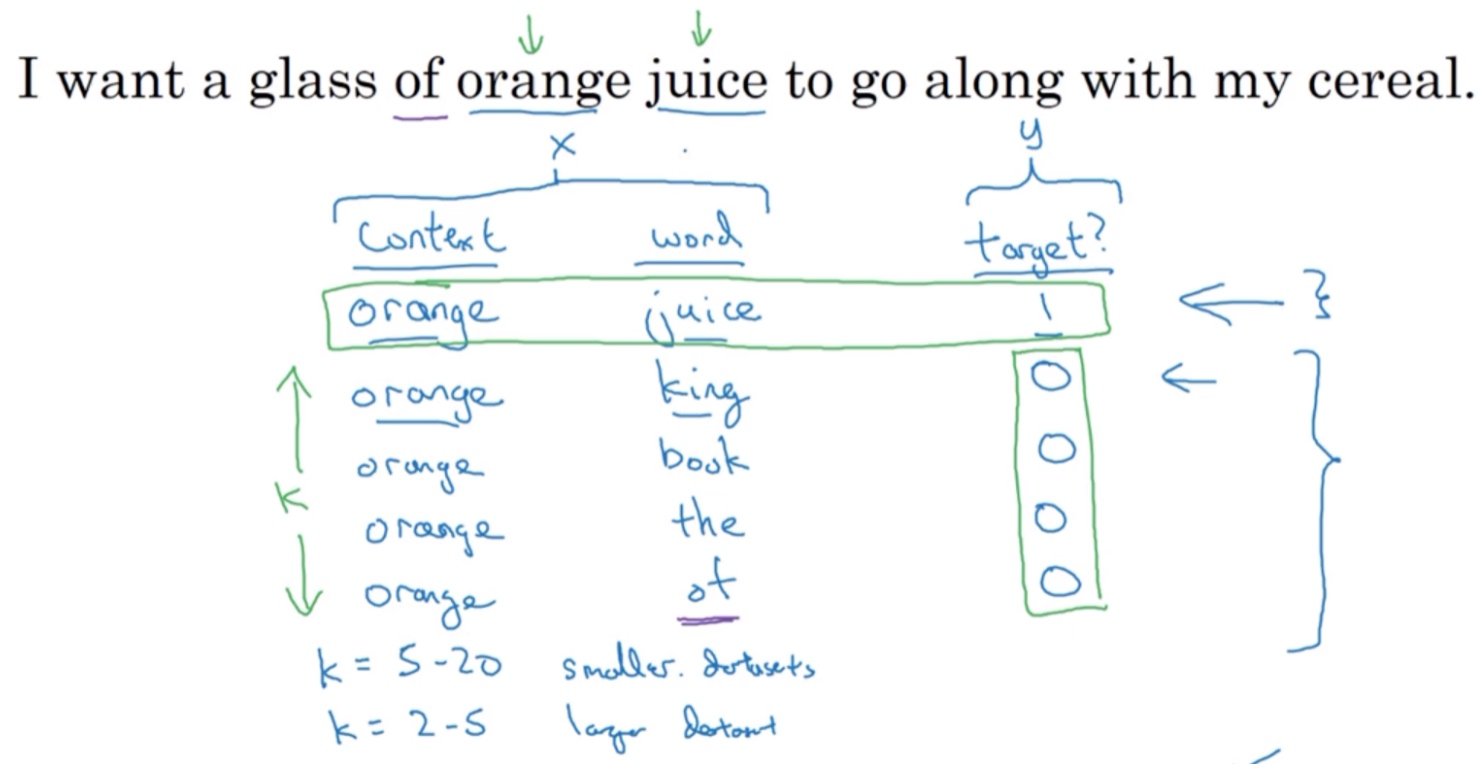Negative sampling是另外一种有效的求解embedding matrix E的方法. 它的做法是判断选取的context word和target word是否构成一组正确的context-target对, 一般包含一个正样本和k个负样本. 例如, ‘orange’为context word，’juice’为target word, 很明显’orange juice’是一组context-target对，为正样本，相应的target label为1. 若’orange’为context word不变，target word随机选择’king, book, the, of’等. 这些都不是正确的context-target对，为负样本，相应的target label为0。一般地，固定某个context word对应的负样本个数k一般遵循:

• 若训练样本较小，k一般选择5～20
• 若训练样本较大，k一般选择2～5即可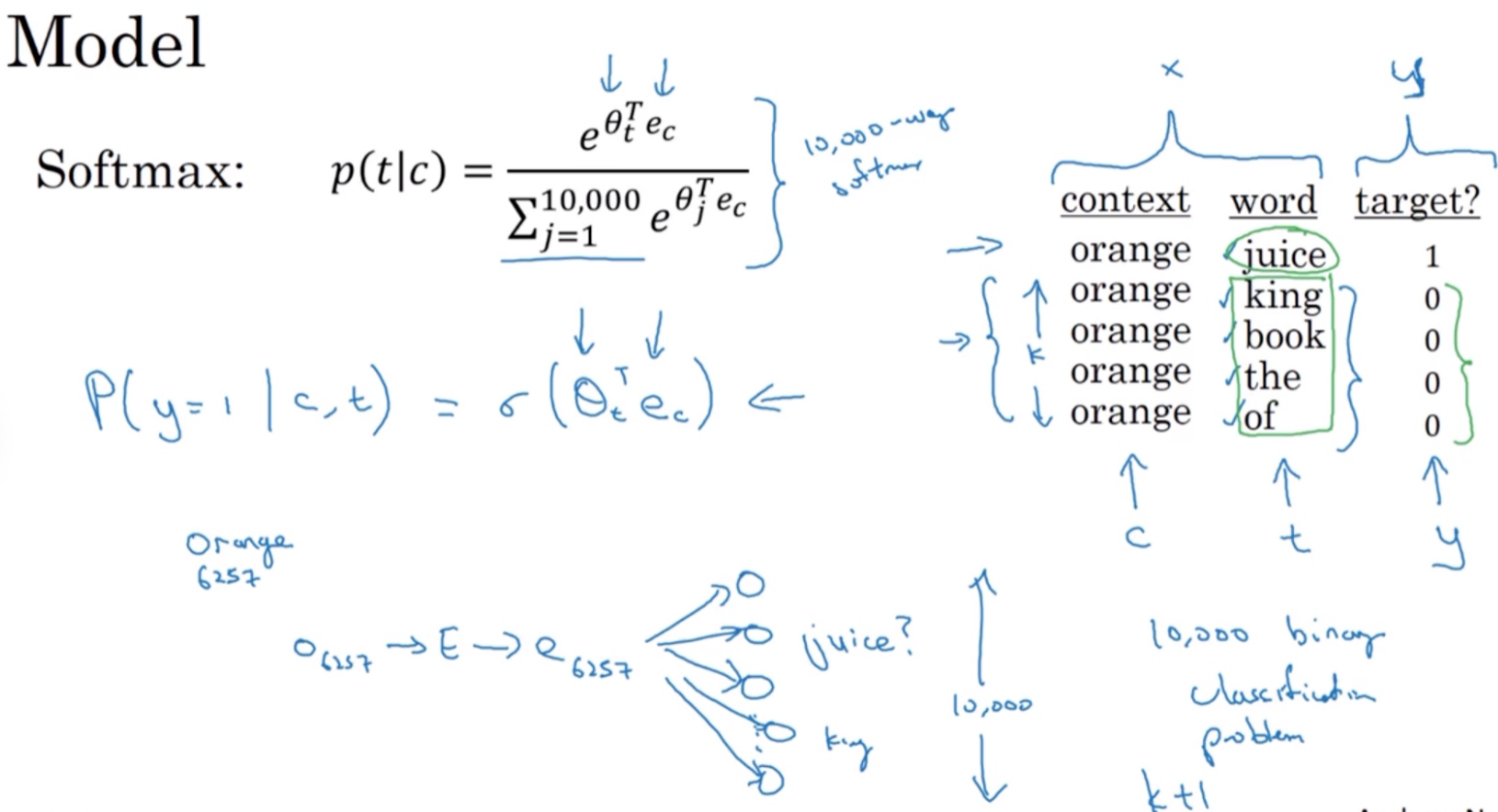Negative sampling的数学模型为：

$P(y=1|c,t)=\sigma(\theta^T_t\cdot e_c)$ 其中，$\sigma$表示sigmoid激活函数, $\theta_t$是参数向量, $e_c$是上下文词的嵌入向量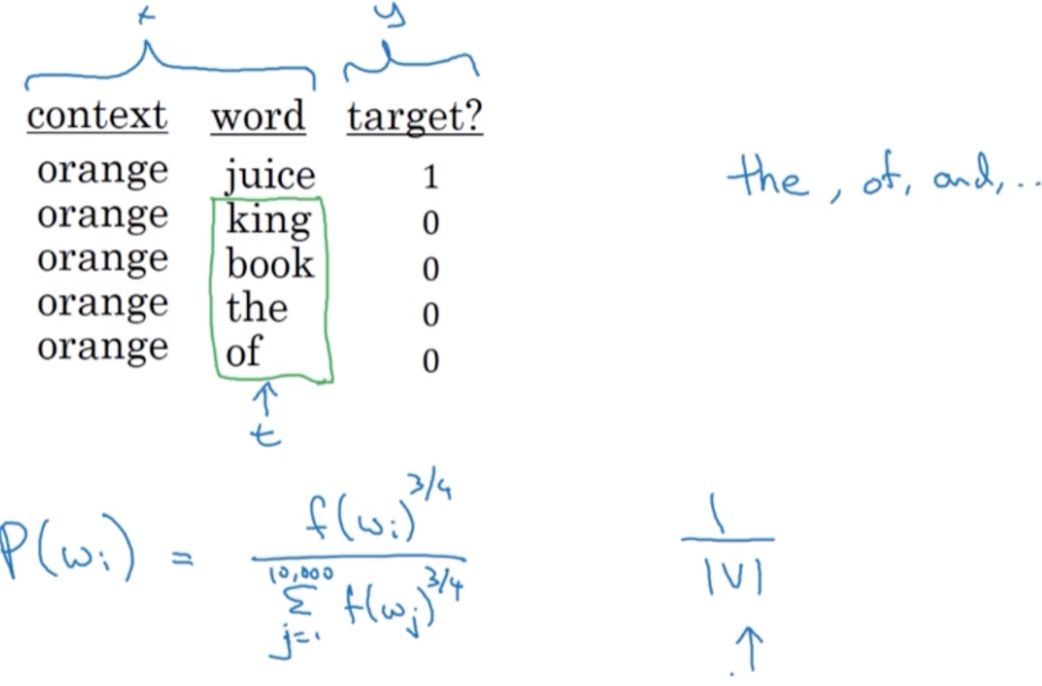$P(w_i)=\frac{f(w_i)^{\frac34}}{\sum_j^{10000}f(w_j)^{\frac34}}$ 其中，$f(w_i)$表示单词$w_i$在单词表中出现的概率(词频),通过$\frac{3}{4}$次方的计算，使其处于完全独立的分布和训练集的观测分布两个极端之间

## GloVe 词向量（GloVe Word Vectors）

[Pennington J, Socher R, Manning C. Glove: Global Vectors for Word Representation[C]// Conference on Empirical Methods in Natural Language Processing. 2014:1532-1543.]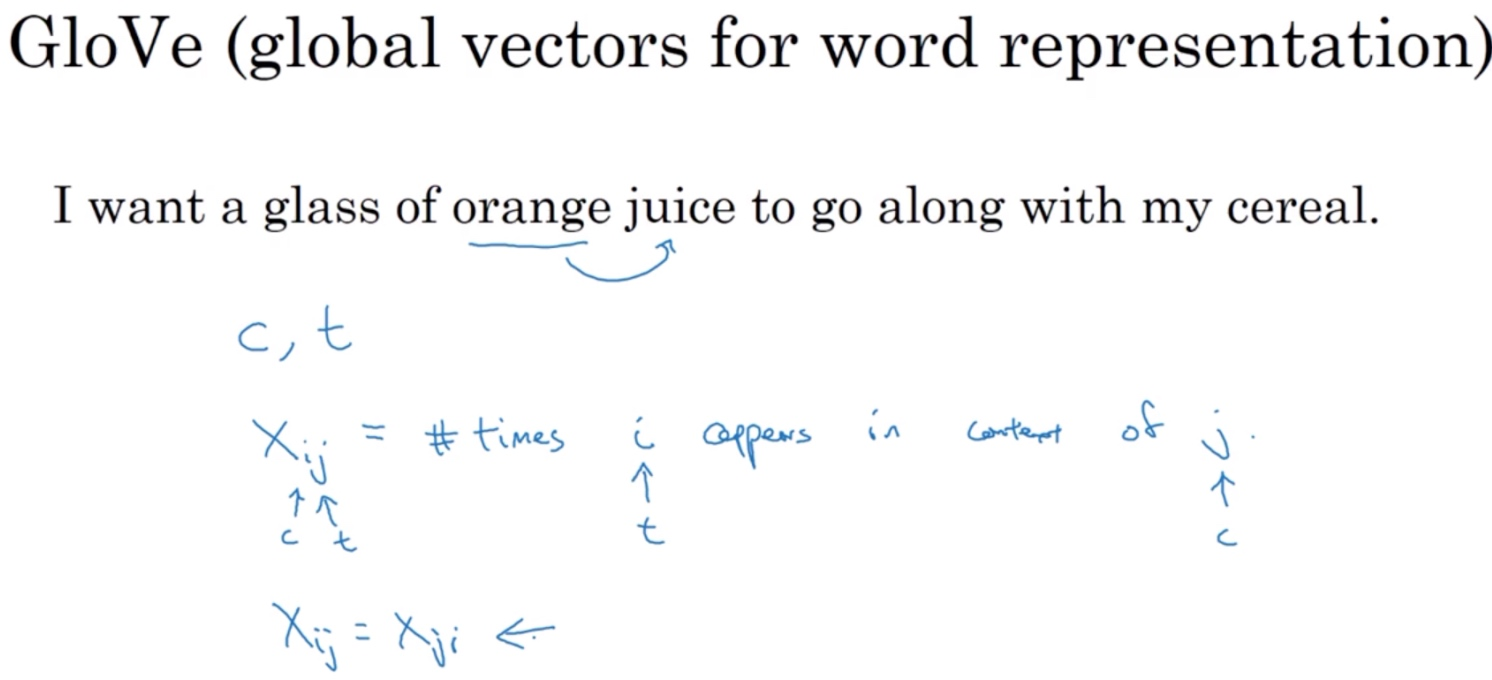GloVe算法引入了一个新的参数:

• $X_{ij}$: 表示单词i出现在单词j之前的次数, 即i和j同时出现的次数
• 其中，i表示context，j表示target
• 一般地，如果不限定context一定在target的前面，则有对称关系$X_{ij}=X_{ji}$
• 如果有限定先后，则$X_{ij}\neq X_{ji}$接下来的讨论中，我们默认存在对称关系$X_{ij}=X_{ji}$
• ($X_{ij}$就是一个能够获取单词i和单词j出现位置相近时或是彼此接近的频率的计数器)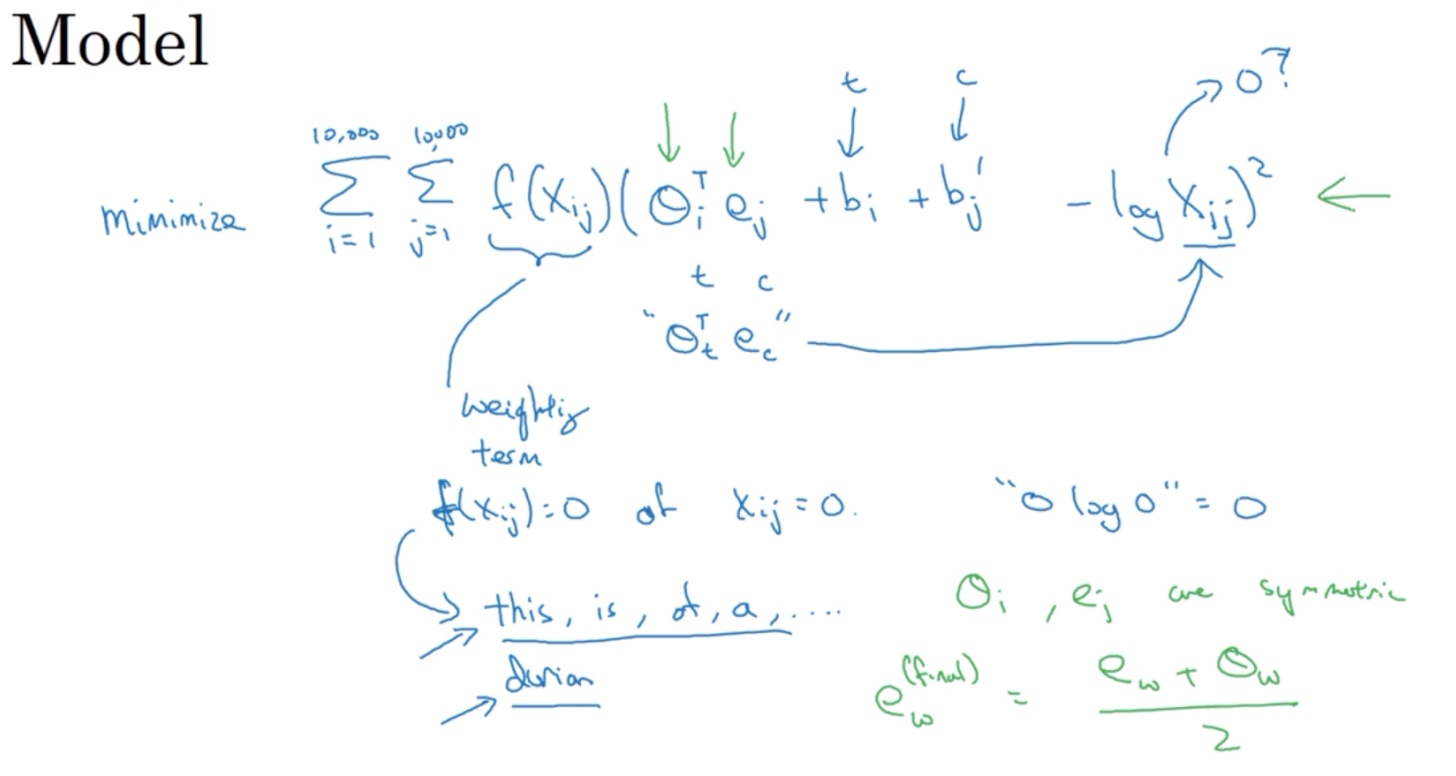GloVe模型的loss function为:

$L=\sum_{i=1}^{10000}\sum_{j=1}^{10000}(\theta_i^Te_j-log X_{ij})^2$

$$L=\sum_{i=1}^{10000}\sum_{j=1}^{10000}f(X_{ij})(\theta_i^Te_j-log X_{ij})^2$$

$X_{ij}=0$时，权重因子$f(X_{ij})=0$. (即$0log0= 0$，这个的意思是如果$X_{ij}=0$，先不要进行求和，所以这个’log 0’项就是不相关项). 这种做法直接忽略了无任何相关性的context和target，只考虑$X_{ij} \gt 0$的情况.

$$L=\sum_{i=1}^{10000}\sum_{j=1}^{10000}f(X_{ij})(\theta_i^Te_j+b_i+b_j’-log X_{ij})^2$$

$$e_w^{final}=\frac{e_w+\theta_w}{2}$$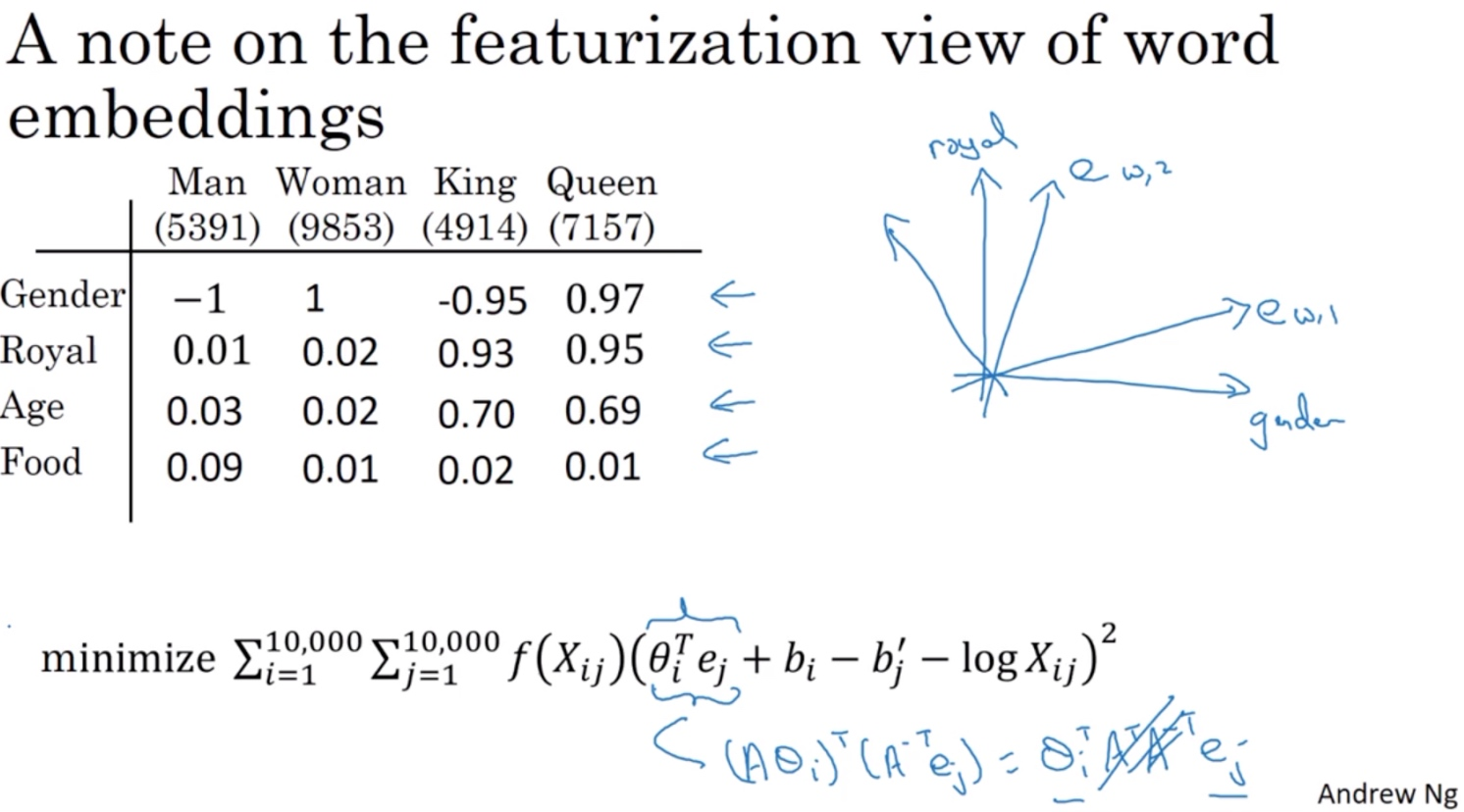## 情感分类（Sentiment Classification）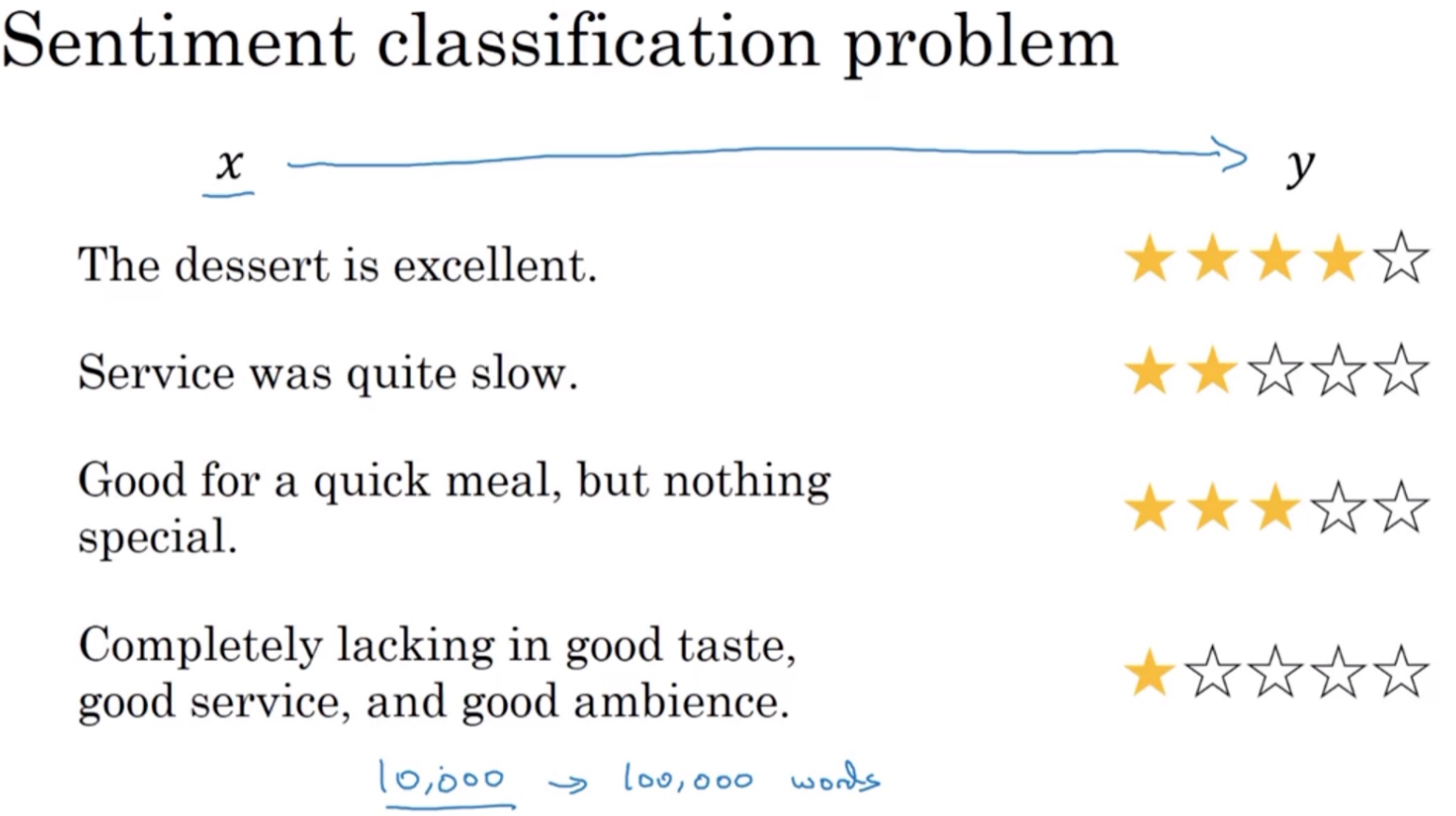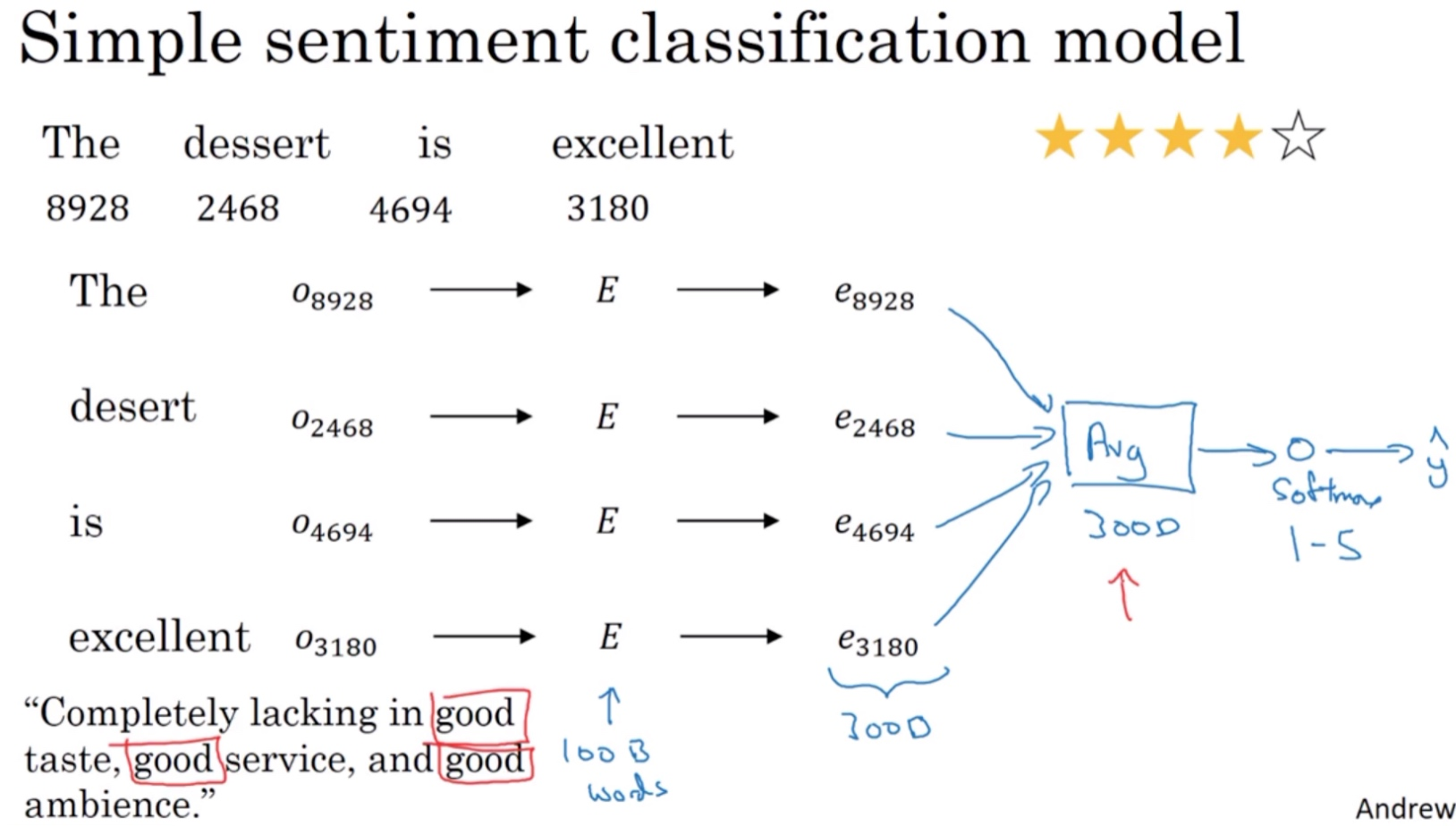• Completely lacking in good taste, good service, and good ambience.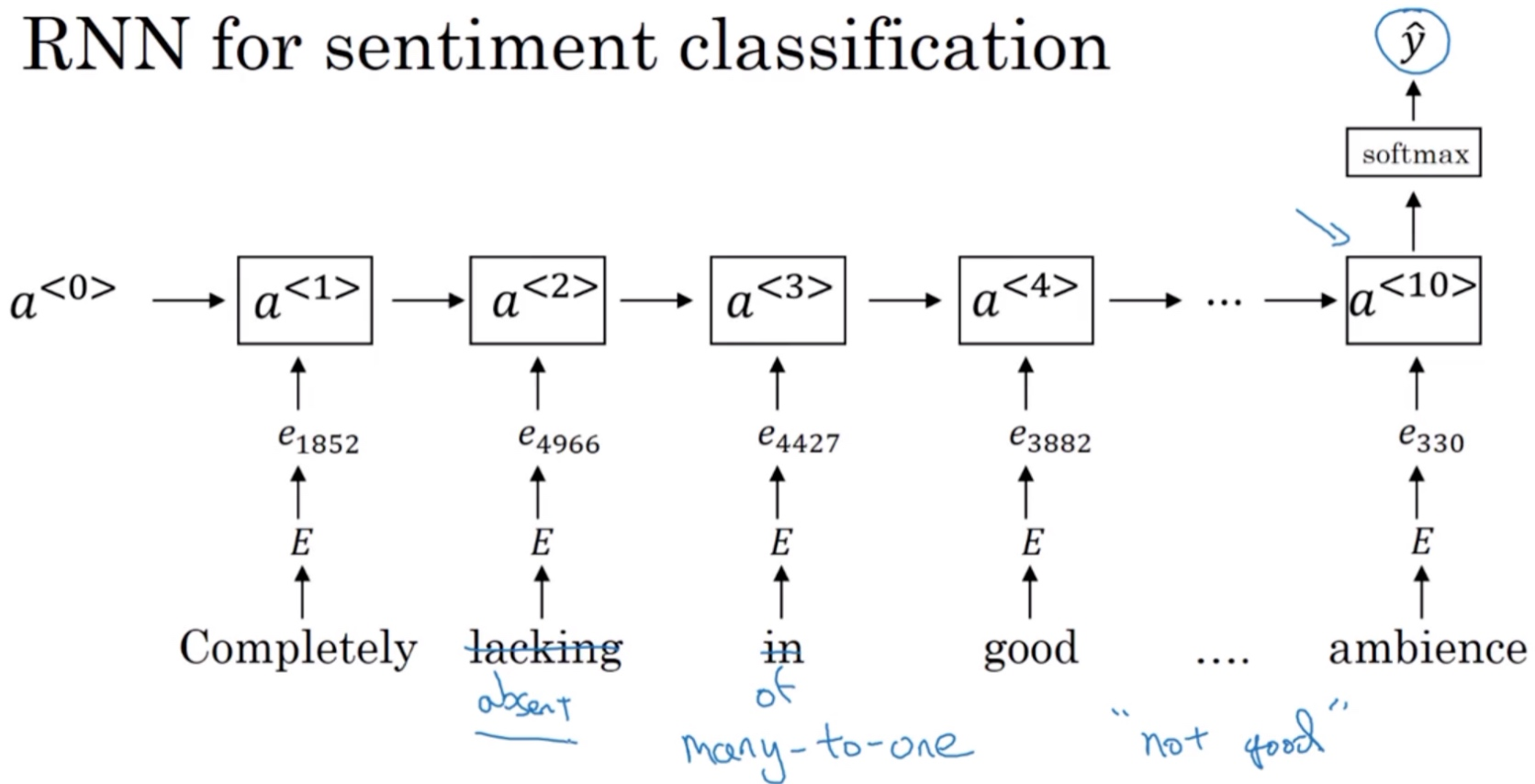## 词嵌入除偏（Debiasing Word Embeddings）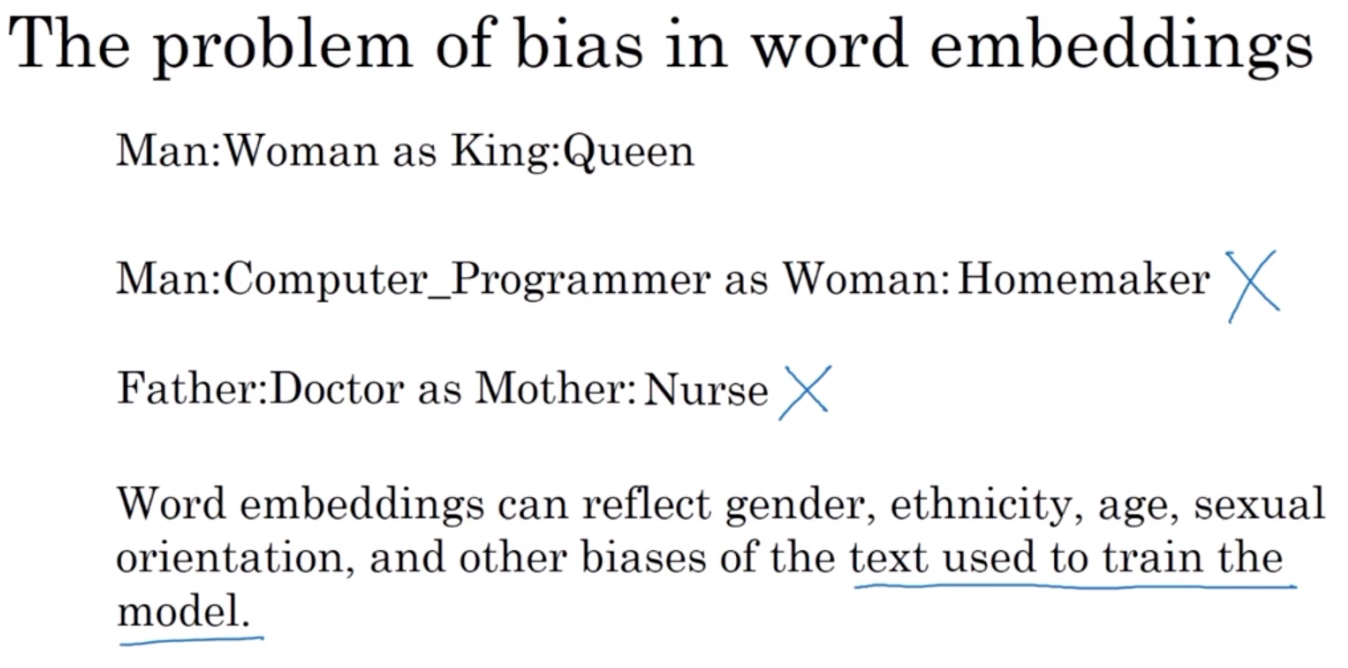Word embeddings中存在一些性别、宗教、种族等偏见(bias)或者歧视. 例如下面这3句话：

• Man: Woman as King: Queen
• Man: Computer programmer as Woman: Homemaker
• Father: Doctor as Mother: Nurse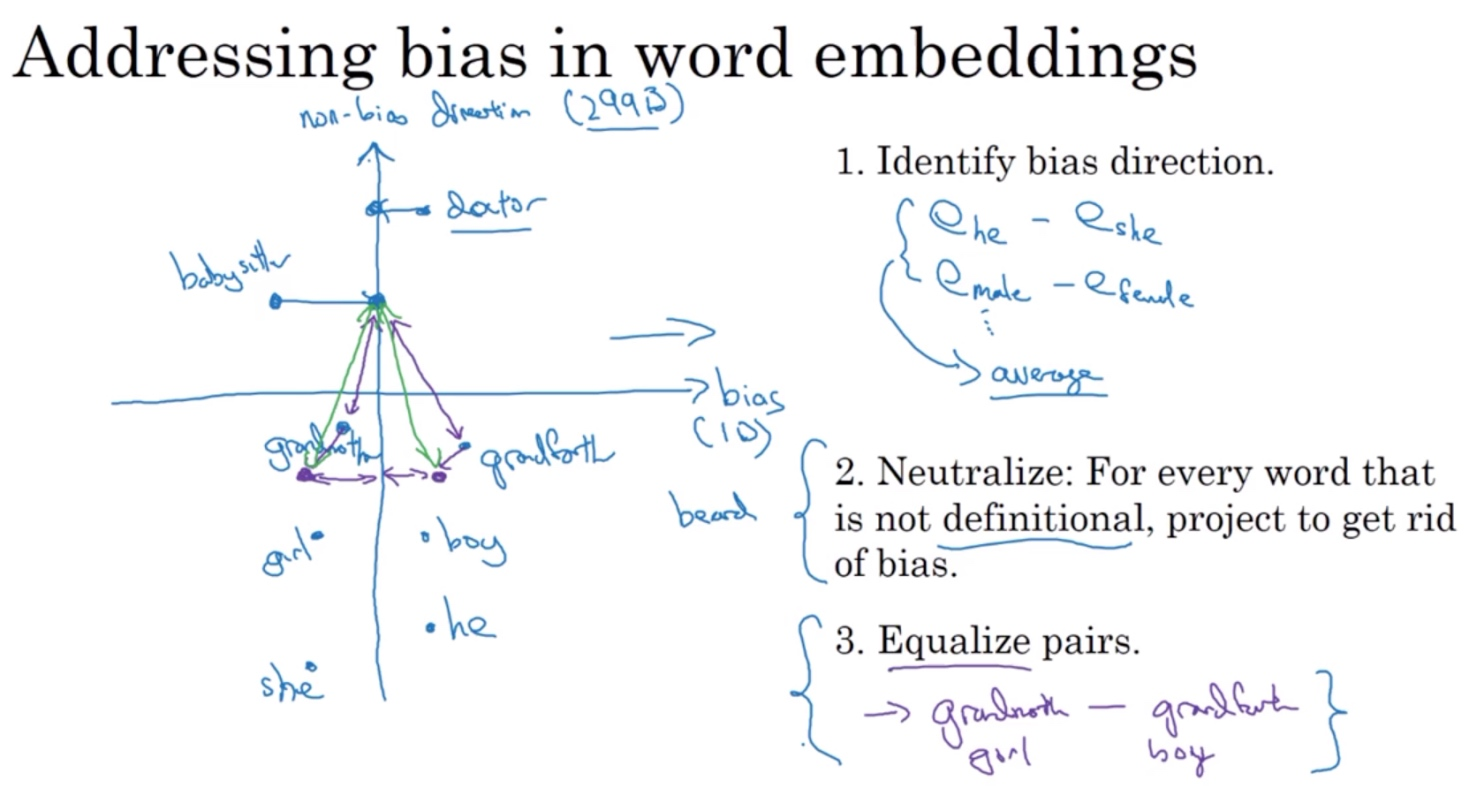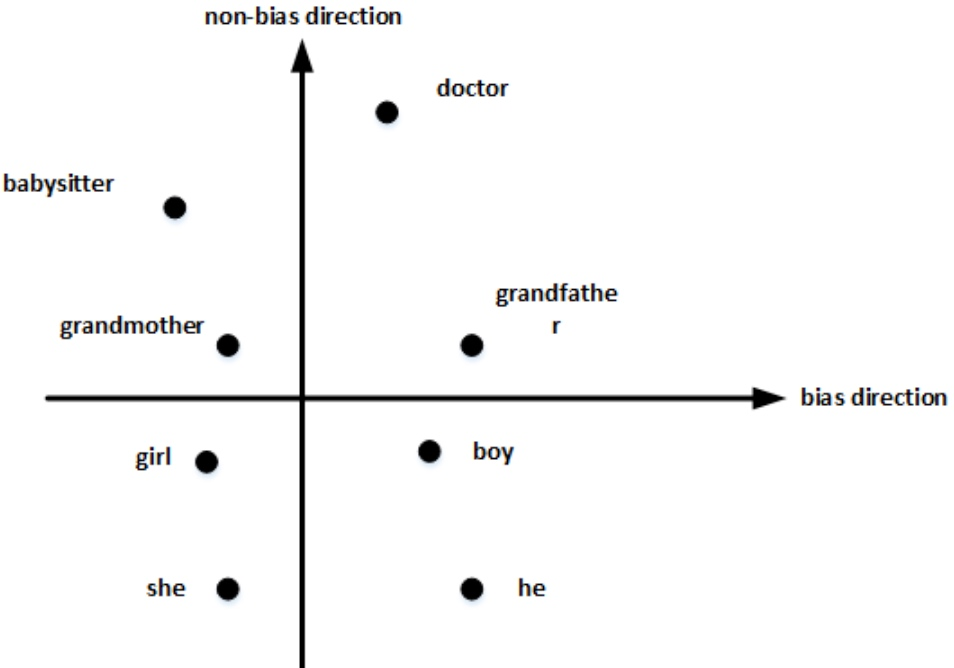$$bias\ direction=\frac1N ((e_{he}-e_{she})+(e_{male}-e_{female})+\cdots)$$